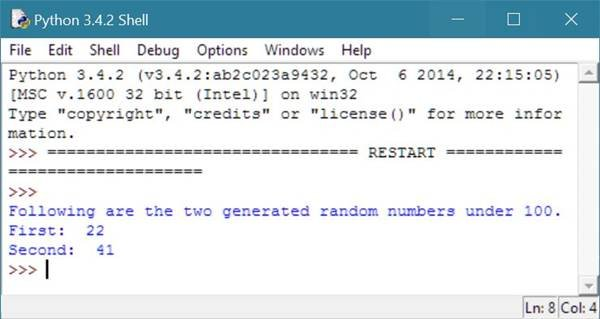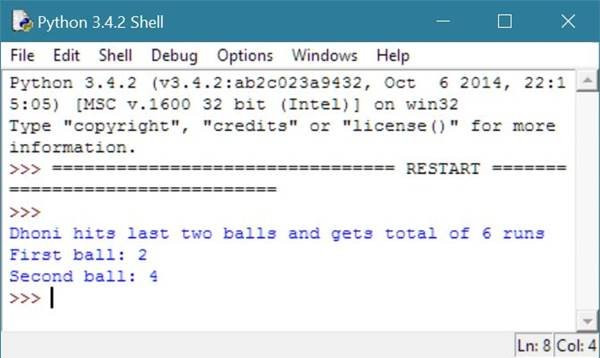# Python import Statement

Here you will learn about import statement in Python with example.

Usually, import statement in Python is the first statement or first line of the program.

The import statement is used to import or load modules (modules are files, contains code to be used in other program just by importing that module using the import statement), for example, to load random module, follow the below general form.

`import random`

## Python import Statement Example

Let's take an example of import statement in Python.

```# Python import Statement Example
import random
val1 = random.randrange(100);
val2 = random.randrange(100);
print("Following are the two generated random numbers \
under 100.");
print("First: ",val1);
print("Second: ",val2);```

Here is the sample output produced by the above import statement example code in Python.As you can notice from the above example and its output, random module contains a function named randrange() used in the above example. The function randrange() in Python produces a random integer value. You can provide a value in the bracket to generate random numbers within limit.

You can also notice from the above example, the function randrange() doesn't called directly, rather called with random.randrange() just because the above program accesses the function named randrange() through its module named random.

Let's take another example of python import statement.

```# Python import Statement Example
import random
first_ball_run = random.randrange(6)+1;
second_ball_run = random.randrange(6)+1;
total_run = first_ball_run + second_ball_run;
print("Dhoni hits last two balls and gets total of",total_run,"runs");
print("First ball:",first_ball_run);
print("Second ball:",second_ball_run);```

Here is the sample output produced by the above Python import statement example.Tools
Calculator